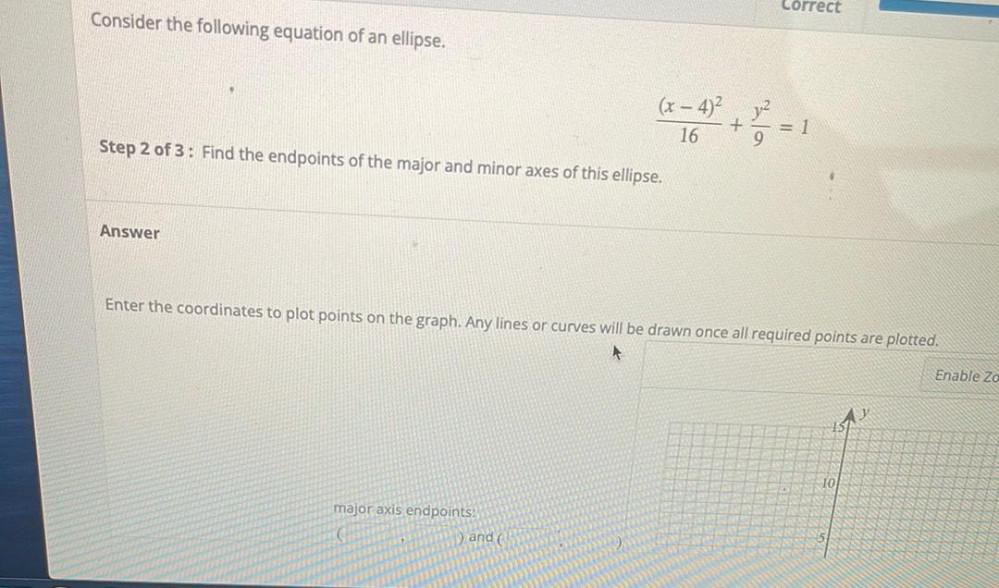Question:

# Correct Consider the following equation of an ellipse. (x – 4) jul + = 1 16 Step 2 of 3: Find the endpoints of the major and minCorrect Consider the following equation of an ellipse. (x – 4) jul + = 1 16 Step 2 of 3: Find the endpoints of the major and minor axes of this ellipse. Answer Enter the coordinates to plot points on the graph. Any lines or curves will be drawn once all required points are plotted. Enable Zo 10 major axis endpoints: ) and